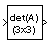# Determinant of 3x3 Matrix

Compute determinant of matrix

• Library:
• Aerospace Blockset / Utilities / Math Operations

•## Description

The Determinant of 3x3 Matrix block computes the determinant for the input matrix. For related equations, see Algorithms.

## Ports

### Input

expand all

Input matrix, specified as a 3-by-3 matrix.

Data Types: `double`

### Output

expand all

Determinant, output as a scalar.

Data Types: `double`

## Algorithms

The input matrix has the form of

`$A=\left[\begin{array}{ccc}{A}_{11}& {A}_{12}& {A}_{13}\\ {A}_{21}& {A}_{22}& {A}_{23}\\ {A}_{31}& {A}_{32}& {A}_{33}\end{array}\right]$`

The determinant of the matrix has the form of

`$det\left(A\right)={A}_{11}\left({A}_{22}{A}_{33}-{A}_{23}{A}_{32}\right)-{A}_{12}\left({A}_{21}{A}_{33}-{A}_{23}{A}_{31}\right)+{A}_{13}\left({A}_{21}{A}_{32}-{A}_{22}{A}_{31}\right)$`

## Version History

Introduced before R2006a